# ELECTROMAGNETISM WHAT IS ELECTROMAGNETISM ELECTROMAGNETISM IS NOTHING BUT

• Slides: 18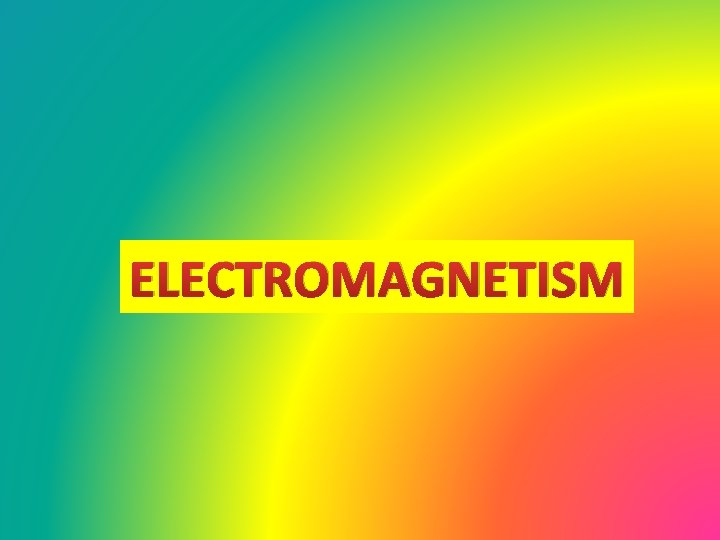ELECTROMAGNETISM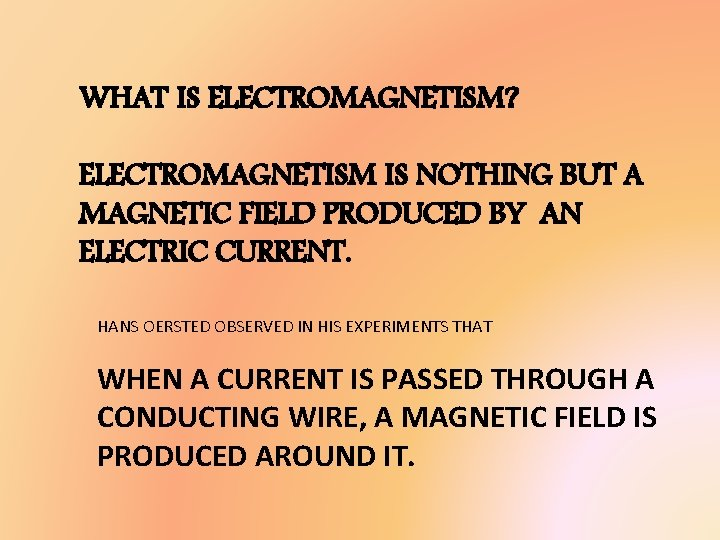WHAT IS ELECTROMAGNETISM? ELECTROMAGNETISM IS NOTHING BUT A MAGNETIC FIELD PRODUCED BY AN ELECTRIC CURRENT. HANS OERSTED OBSERVED IN HIS EXPERIMENTS THAT WHEN A CURRENT IS PASSED THROUGH A CONDUCTING WIRE, A MAGNETIC FIELD IS PRODUCED AROUND IT.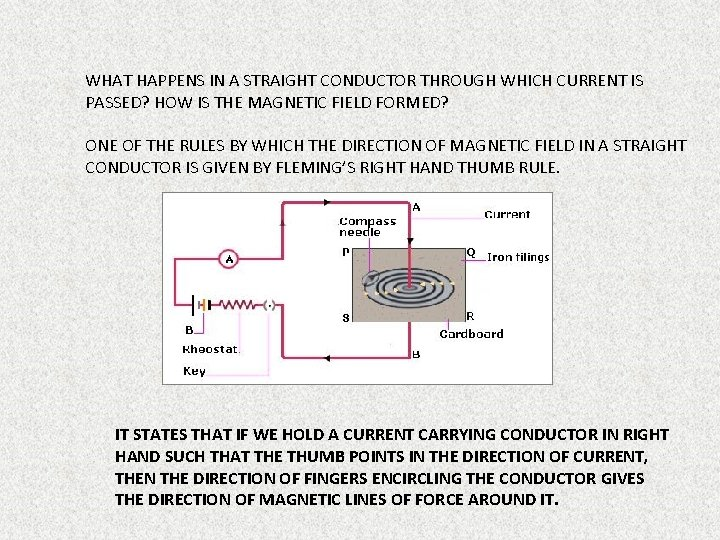WHAT HAPPENS IN A STRAIGHT CONDUCTOR THROUGH WHICH CURRENT IS PASSED? HOW IS THE MAGNETIC FIELD FORMED? ONE OF THE RULES BY WHICH THE DIRECTION OF MAGNETIC FIELD IN A STRAIGHT CONDUCTOR IS GIVEN BY FLEMING’S RIGHT HAND THUMB RULE. IT STATES THAT IF WE HOLD A CURRENT CARRYING CONDUCTOR IN RIGHT HAND SUCH THAT THE THUMB POINTS IN THE DIRECTION OF CURRENT, THEN THE DIRECTION OF FINGERS ENCIRCLING THE CONDUCTOR GIVES THE DIRECTION OF MAGNETIC LINES OF FORCE AROUND IT.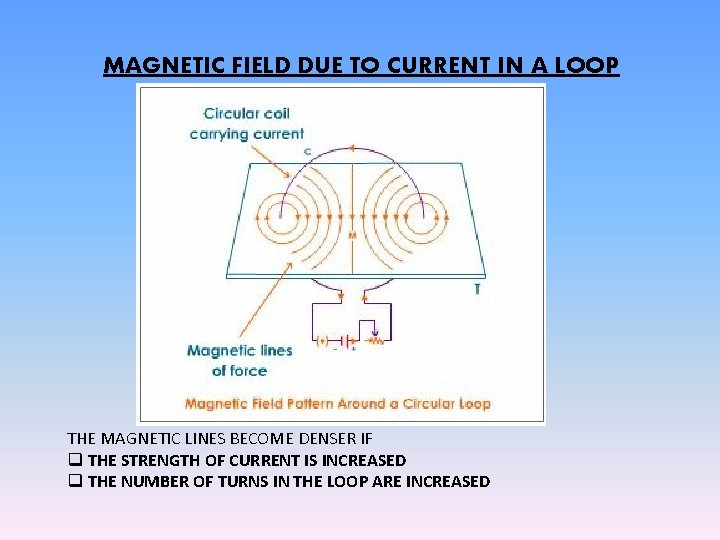MAGNETIC FIELD DUE TO CURRENT IN A LOOP THE MAGNETIC LINES BECOME DENSER IF q THE STRENGTH OF CURRENT IS INCREASED q THE NUMBER OF TURNS IN THE LOOP ARE INCREASED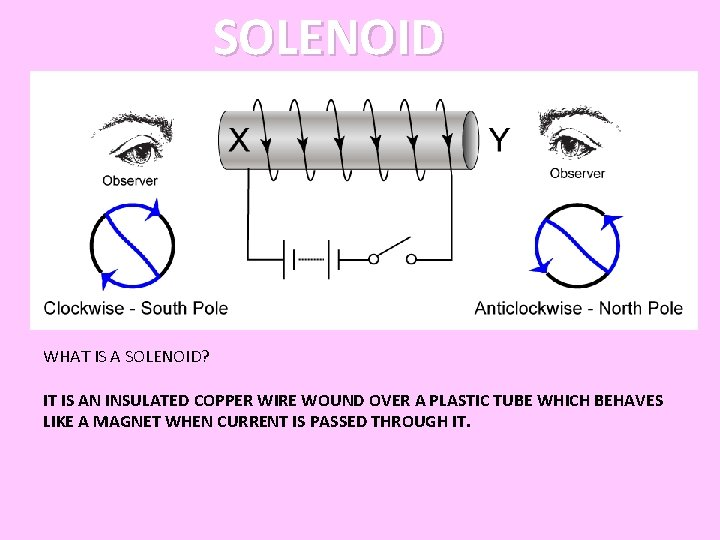SOLENOID WHAT IS A SOLENOID? IT IS AN INSULATED COPPER WIRE WOUND OVER A PLASTIC TUBE WHICH BEHAVES LIKE A MAGNET WHEN CURRENT IS PASSED THROUGH IT.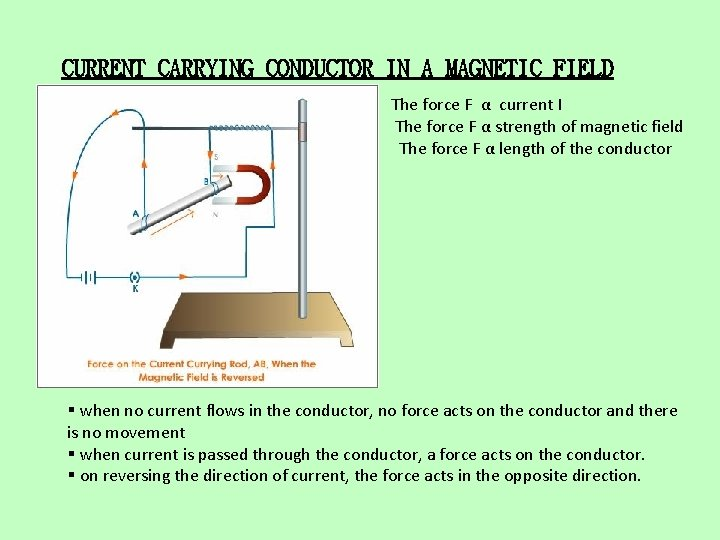CURRENT CARRYING CONDUCTOR IN A MAGNETIC FIELD The force F α current I The force F α strength of magnetic field The force F α length of the conductor § when no current flows in the conductor, no force acts on the conductor and there is no movement § when current is passed through the conductor, a force acts on the conductor. § on reversing the direction of current, the force acts in the opposite direction.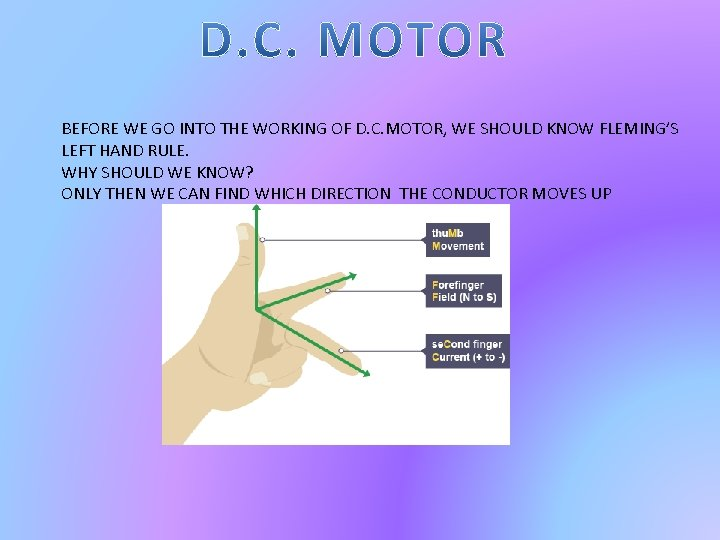BEFORE WE GO INTO THE WORKING OF D. C. MOTOR, WE SHOULD KNOW FLEMING’S LEFT HAND RULE. WHY SHOULD WE KNOW? ONLY THEN WE CAN FIND WHICH DIRECTION THE CONDUCTOR MOVES UPPARTS OF A D. C. MOTOR q CORE: Soft iron laminated cylinder q COIL : Insulated copper wire of many turns q COMMUTATOR: Reverses the direction of the coil every half turn. q BRUSHES: To supply a continuous current to the coil through the commutator q ELECTRO MAGNET: To provide a strong uniform magnetic field q A D. C. SOURCE (BATTERY): To provide current to the coil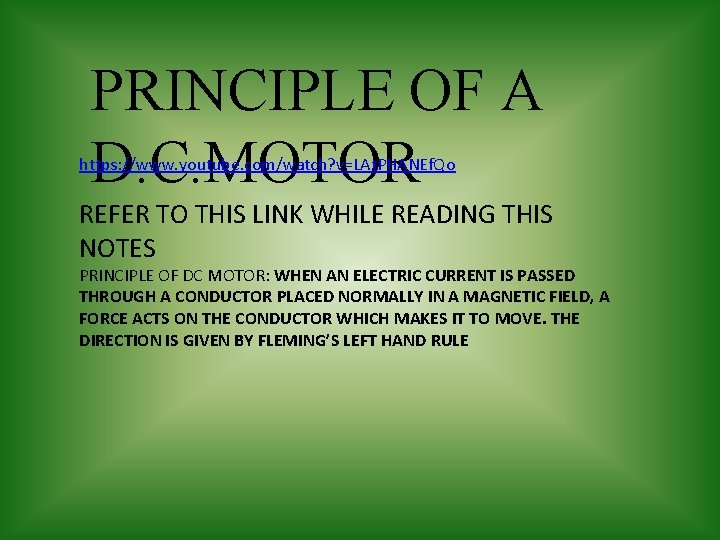PRINCIPLE OF A D. C. MOTOR https: //www. youtube. com/watch? v=LAt. PHANEf. Qo REFER TO THIS LINK WHILE READING THIS NOTES PRINCIPLE OF DC MOTOR: WHEN AN ELECTRIC CURRENT IS PASSED THROUGH A CONDUCTOR PLACED NORMALLY IN A MAGNETIC FIELD, A FORCE ACTS ON THE CONDUCTOR WHICH MAKES IT TO MOVE. THE DIRECTION IS GIVEN BY FLEMING’S LEFT HAND RULEWORKING OF A D. C. MOTOR Ø THE PLANE ABCD IS HORIZONTAL AND SPLIT RING S 1 TOUCHES BRUSH B 1, SPLIT RING S 2 TOUCHES BRUSH B 2 Ø CURRENT FLOWS IN DIRECTION ABCD Ø CURRENT IN ARMS AB AND CD IS PERPENDICULAR TO THE MAGNETIC FIELD. SO EACH ARM EXPERIENCES A FORCE WHICH PRODUCES A COUPLE Ø AS PER FLEMING’S LEFT HAND RULE, AB MOVES DOWNWARDS, AND CD MOVES UPWARDS. (VERY IMPORTANT) Ø COIL CD MOVES UPWARDS, Ø 90 DEGREES- ---- PERPENDICULAR TO THE PLANE OF PAPER. SO NO CONTACT BETWEEN BRUSH AND SPLIT RING. SO CURRENT FLOWS Ø 180 DEGREES ---- ROTATES DUE TO MOMENTUM. SO SPLIT RING S 1 TOUCHES BRUSH B 2, SPLIT RING S 2 TOUCHES BRUSH B 1. NOW FORCE IS MAXIMUM WHEN THE COIL TURNS 180 DEGREES Ø 270 DEGREES ---- AGAIN NO CONTACT LIKE IN 90 DEGREES. BUT DUE TO MOMENTUM IT ROTATES Ø 360 DEGREES ---- CONTINUES TO MOVE IN THE CLOCKWISE DIRECTION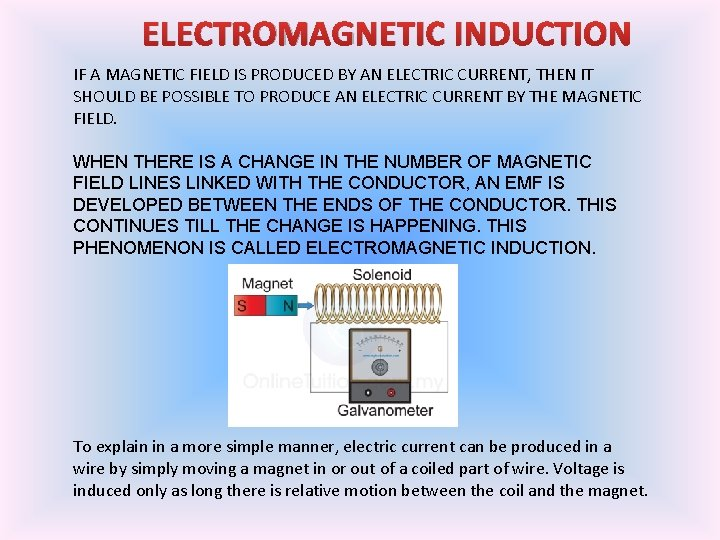ELECTROMAGNETIC INDUCTION IF A MAGNETIC FIELD IS PRODUCED BY AN ELECTRIC CURRENT, THEN IT SHOULD BE POSSIBLE TO PRODUCE AN ELECTRIC CURRENT BY THE MAGNETIC FIELD. WHEN THERE IS A CHANGE IN THE NUMBER OF MAGNETIC FIELD LINES LINKED WITH THE CONDUCTOR, AN EMF IS DEVELOPED BETWEEN THE ENDS OF THE CONDUCTOR. THIS CONTINUES TILL THE CHANGE IS HAPPENING. THIS PHENOMENON IS CALLED ELECTROMAGNETIC INDUCTION. To explain in a more simple manner, electric current can be produced in a wire by simply moving a magnet in or out of a coiled part of wire. Voltage is induced only as long there is relative motion between the coil and the magnet.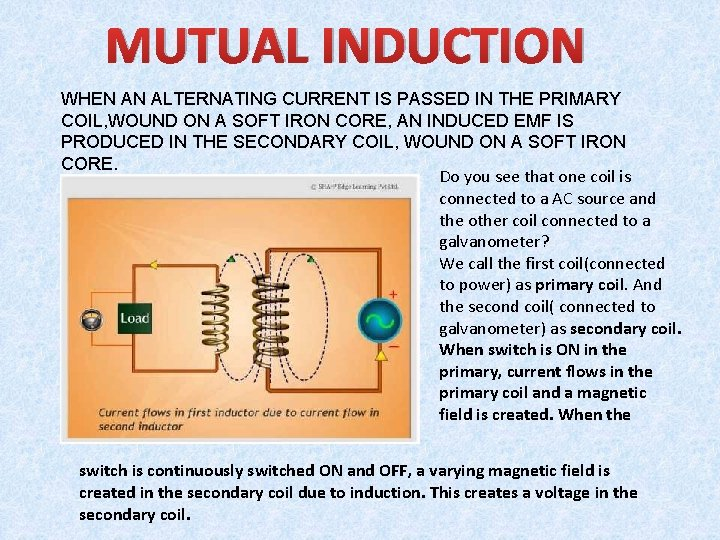MUTUAL INDUCTION WHEN AN ALTERNATING CURRENT IS PASSED IN THE PRIMARY COIL, WOUND ON A SOFT IRON CORE, AN INDUCED EMF IS PRODUCED IN THE SECONDARY COIL, WOUND ON A SOFT IRON CORE. Do you see that one coil is connected to a AC source and the other coil connected to a galvanometer? We call the first coil(connected to power) as primary coil. And the second coil( connected to galvanometer) as secondary coil. When switch is ON in the primary, current flows in the primary coil and a magnetic field is created. When the switch is continuously switched ON and OFF, a varying magnetic field is created in the secondary coil due to induction. This creates a voltage in the secondary coil.PRINCIPLE OF A TRANSFORMERS WORK ON THE PRINCIPLE OF MUTUAL INDUCTION. TRANSFORMER IS A DEVICE WHERE THE AMPLITUDE OF AN ALTERNATING EMF CAN BE EITHER INCREASED OR DECREASED. HOWEVER THE FREQUENCY IS UNCHANGED (50 Hz) TRANSFORMERS STEP UP TRANSFORMERS 1. To change from low to high voltage 2. Number of turns of sec. coil is more compared to primary STEP DOWN TRANSFORMERS to change from high voltage to low voltage Number of turns of primary coil is more compared to primaryConstruction of a transformer TURNS RATIO: NUMBER OF TURNS IN SECONDARY COIL NUMBER OF TURNS IN PRIMARY COIL IF THIS RATIO> 1, THEN IT IS A STEP UP TRNASFORMER IF THIS RATIO< 1, THEN IT IS A STEP DOWN TRNASFORMER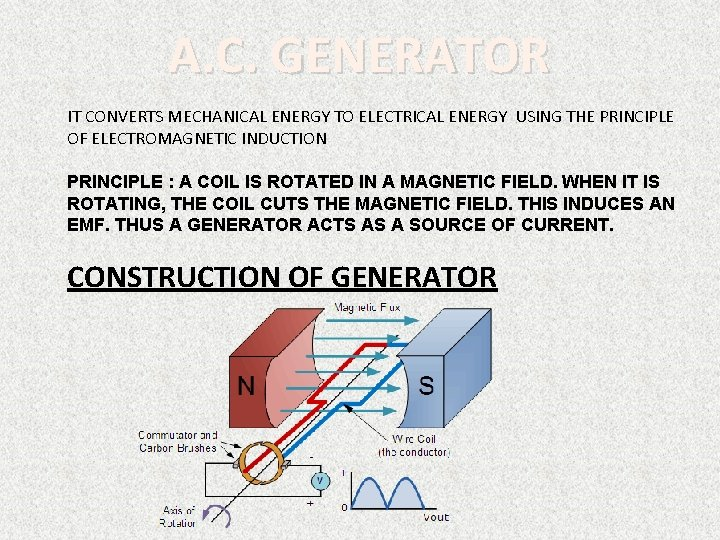A. C. GENERATOR IT CONVERTS MECHANICAL ENERGY TO ELECTRICAL ENERGY USING THE PRINCIPLE OF ELECTROMAGNETIC INDUCTION PRINCIPLE : A COIL IS ROTATED IN A MAGNETIC FIELD. WHEN IT IS ROTATING, THE COIL CUTS THE MAGNETIC FIELD. THIS INDUCES AN EMF. THUS A GENERATOR ACTS AS A SOURCE OF CURRENT. CONSTRUCTION OF GENERATOR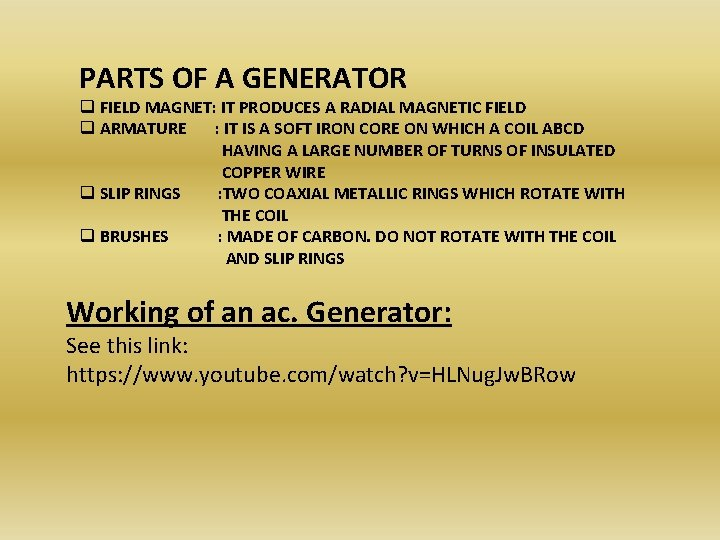PARTS OF A GENERATOR q FIELD MAGNET: IT PRODUCES A RADIAL MAGNETIC FIELD q ARMATURE : IT IS A SOFT IRON CORE ON WHICH A COIL ABCD HAVING A LARGE NUMBER OF TURNS OF INSULATED COPPER WIRE q SLIP RINGS : TWO COAXIAL METALLIC RINGS WHICH ROTATE WITH THE COIL q BRUSHES : MADE OF CARBON. DO NOT ROTATE WITH THE COIL AND SLIP RINGS Working of an ac. Generator: See this link: https: //www. youtube. com/watch? v=HLNug. Jw. BRow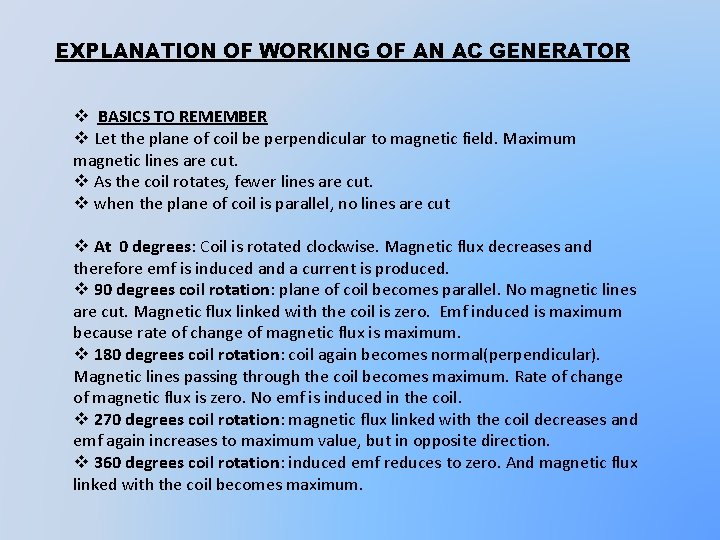EXPLANATION OF WORKING OF AN AC GENERATOR v BASICS TO REMEMBER v Let the plane of coil be perpendicular to magnetic field. Maximum magnetic lines are cut. v As the coil rotates, fewer lines are cut. v when the plane of coil is parallel, no lines are cut v At 0 degrees: Coil is rotated clockwise. Magnetic flux decreases and therefore emf is induced and a current is produced. v 90 degrees coil rotation: plane of coil becomes parallel. No magnetic lines are cut. Magnetic flux linked with the coil is zero. Emf induced is maximum because rate of change of magnetic flux is maximum. v 180 degrees coil rotation: coil again becomes normal(perpendicular). Magnetic lines passing through the coil becomes maximum. Rate of change of magnetic flux is zero. No emf is induced in the coil. v 270 degrees coil rotation: magnetic flux linked with the coil decreases and emf again increases to maximum value, but in opposite direction. v 360 degrees coil rotation: induced emf reduces to zero. And magnetic flux linked with the coil becomes maximum.THANK YOU FOR YOUR PATIENCE